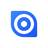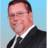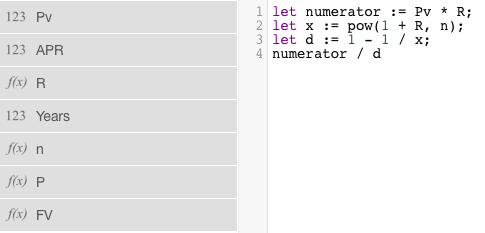Financial calculations in formula?

Is there a plan to offer financial calculations in the formula fields ie; PMT, FV, etc. Thanks!!Hi,

At the moment there are no specific plans for the predefined calculations like PMT or FV, but I have added it now in our feature request list.

Best, Jörg

That's awesome. I have used FileMaker for over 30 years in real estate and the calculation fields would be amazing to have. I have tried and failed at trying to possible layout a formula but I just don't have the chops like you guys do. Please see what you can do to make this happed as it would be awesome. Of course the big question is how will I import tons of records that have a been created but that discusiion is for another day.Scotty ... here ya go.. using this site

https://superuser.com/questions/871404/what-would-be-the-the-mathematical-equivalent-of-this-excel-formula-pmt

The code would look like this.. .. DISCLAIMER.. I cannot find an exponation function in NX.. so I did it the old school way.. :)

let Pv:= 220000;
let APR := number(4.75);
let R:=(APR/100)/12;
let n := 360;
let numerator := Pv * R;
let x := 1 + R;
for i in range(1, n) do
x := x * (1 + R)
end;
let denominator := 1 - (1 / x);
numerator / denominator

Thus P=1147.62414030685Here is FV = PV x (1 + R)^N

let y := 1 + R;
let x := y;
for i in range(1, n) do
x := x * y
end;
Pv * x

Thank you Mconneen. The pmt function is really the one that I needed. I will give this formula that you referred to a try. Thank you my friend !! My HP 12C makes this so easy. I should have paid better attention in math class...Support replied to an email.. There is a pow(x,y) function for x^y.. so this is a bit cleaner.